# Software Engineering | Critical Path Method

Critical Path Method (CPM) is a method used in project planning, generally for project scheduling for the on-time completion of the project. It actually helps in the determination of the earliest time by which the whole project can be completed. There are two main concepts in this method namely critical task and critical path. Critical task is the task/activity which can’t be delayed otherwise the completion of the whole project will be delayed. It must be completed on-time before starting the other dependent tasks.

Critical path is a sequence of critical tasks/activities and is the largest path in the project network. It gives us the minimum time which is required to complete the whole project. The activities in the critical path are known as critical activities and if these activities are delayed then the completion of the whole project is also delayed.

Major steps of the Critical Path Method:

1. Identifying the activities
2. Construct the project network
3. Perform time estimation using forward and backward pass
4. Identify the critical path

The table given below contains the activity label, its respective duration (in weeks) and its precedents. We will use critical path method to find the critical path and activities of this project.

Activity Duration (in weeks) Precedents
A 6
B 4
C 3 A
D 4 B
E 3 B
F 10
G 3 E, F
H 2 C, D

Rules for designing the Activity-on-Node network diagram:

• A project network should have only one start node
• A project network should have only one end node
• A node has a duration
• Links normally have no duration
• “Precedents” are the immediate preceding activities
• Time moves from left to right in the project network
• A network should not contain loops
• A network should not contain dangles

Node Representation: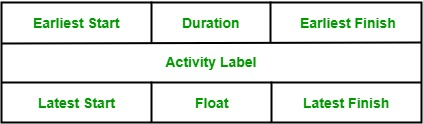• Activity label is the name of the activity represented by that node.
• Earliest Start is the date or time at which the activity can be started at the earliest.
• Earliest Finish is the date or time at which the activity can completed at the earliest.
• Latest Start is the date or time at which the activity can be started at the latest.
• Latest Finish is the date or time at which the activity can be finished at the latest.
• Float is equal to the difference between earliest start and latest start or earliest finish and latest finish.

Activity-On-Node diagram: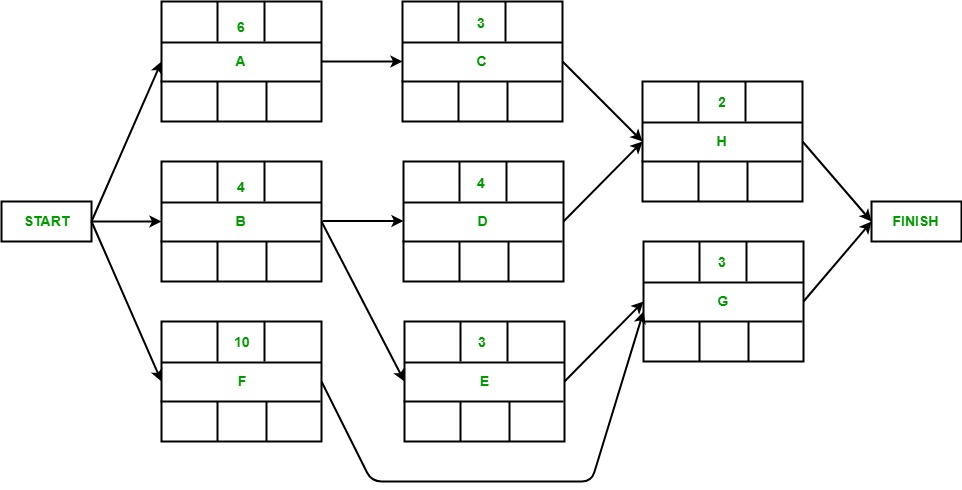Forward Pass:
The forward pass is carried out to calculate the earliest dates on which each activity may be started and completed.

1. Activity A may start immediately. Hence, earliest date for its start is zero i.e. ES(A) = 0. It takes 6 weeks to complete its execution. Hence, earliest it can finish is week 6 i.e. EF(A) = 6.
2. Activity B may start immediately. Hence, earliest date for its start is zero i.e. ES(B) = 0. It takes 4 weeks to complete its execution. Hence, earliest it can finish is week 4 i.e. EF(B) = 4.
3. Activity F may start immediately. Hence, earliest date for its start is zero i.e. ES(F) = 0. It takes 10 weeks to complete its execution. Hence, earliest it can finish is week 10 i.e. EF(F) = 10.
4. Activity C starts as soon as activity A completes its execution. Hence, earliest week it can start its execution is week 6 i.e. ES(C) = 6. It takes 3 weeks to complete its execution. Hence, earliest it can finish is week 9 i.e. EF(C) = 9.
5. Activity D starts as soon as activity B completes its execution. Hence, earliest week it can start its execution is week 4 i.e. ES(D) = 4. It takes 4 weeks to complete its execution. Hence, earliest it can finish is week 8 i.e. EF(D) = 8.
6. Activity E starts as soon as activity B completes its execution. Hence, earliest week it can start its execution is week 4 i.e. ES(E) = 4. It takes 3 weeks to complete its execution. Hence, earliest it can finish is week 7 i.e. EF(E) = 7.
7. Activity G starts as soon as activity E and activity F completes their execution. Since, activity requires the completion of both for starting its execution, we would consider the MAX(ES(E), ES(F)). Hence, earliest week it can start its execution is week 10 i.e. ES(G) = 10. It takes 3 weeks to complete its execution. Hence, earliest it can finish is week 13 i.e. EF(G) = 13.
8. Activity H starts as soon as activity C and activity D completes their execution. Since, activity requires the completion of both for starting its execution, we would consider the MAX(ES(C), ES(D)). Hence, earliest week it can start its execution is week 9 i.e. ES(H) = 9. It takes 2 weeks to complete its execution. Hence, earliest it can finish is week 11 i.e. EF(H) = 11.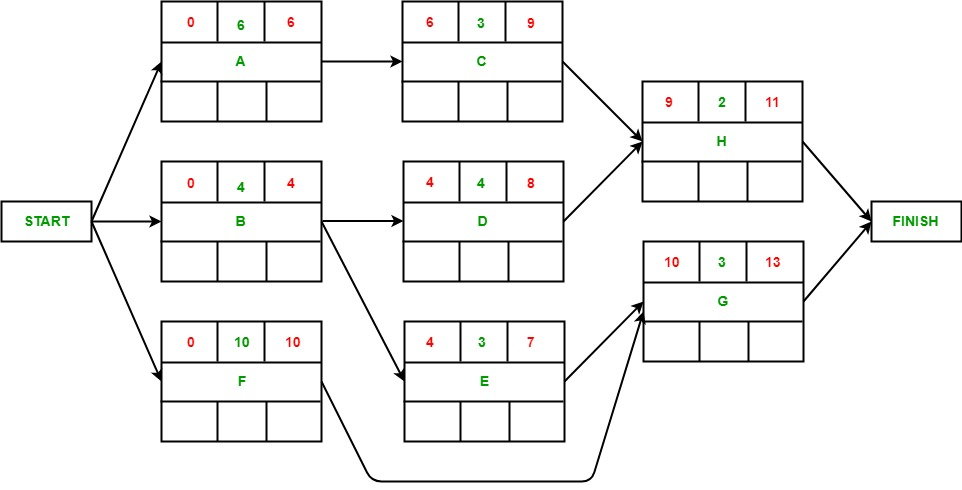Backward Pass:
The backward pass is carried out to calculate the latest dates on which each activity may be started and finished without delaying the end date of the project.
Assumption: Latest finish date = Earliest Finish date (of project).

1. Activity G’s latest finish date is equal to the earliest finish date of the precedent activity of finish according to the assumption i.e. LF(G) = 13. It takes 3 weeks to complete its execution. Hence, latest it can start is week 10 i.e. LS(G) = 10.
2. Activity H’s latest finish date is equal to the earliest finish date of the precedent activity of finish according to the assumption i.e. LF(H) = 13. It takes 2 weeks to complete its execution. Hence, latest it can start is week 11 i.e. LS(H) = 11.
3. The latest end date for activity C would be the latest start date of H i.e. LF(C) = 11. It takes 3 weeks to complete its execution. Hence, latest it can start is week 8 i.e. LS(C) = 8.
4. The latest end date for activity D would be the latest start date of H i.e. LF(D) = 11. It takes 4 weeks to complete its execution. Hence, latest it can start is week 7 i.e. LS(D) = 7.
5. The latest end date for activity E would be the latest start date of G i.e. LF(G) = 10. It takes 3 weeks to complete its execution. Hence, latest it can start is week 7 i.e. LS(E) = 7.
6. The latest end date for activity F would be the latest start date of G i.e. LF(G) = 10. It takes 10 weeks to complete its execution. Hence, latest it can start is week 0 i.e. LS(F) = 0.
7. The latest end date for activity A would be the latest start date of C i.e. LF(A) = 8. It takes 6 weeks to complete its execution. Hence, latest it can start is week 2 i.e. LS(A) = 2.
8. The latest end date for activity B would be the earliest of the latest start date of D and E i.e. LF(B) = 7. It takes 4 weeks to complete its execution. Hence, latest it can start is week 3 i.e. LS(B) = 3.

9.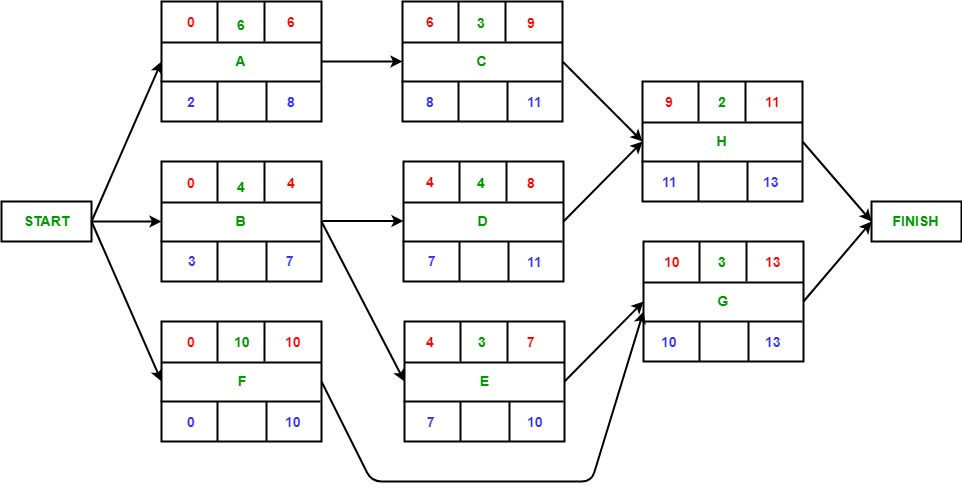Identifying Critical Path:
Critical path is the path which gives us or helps us to estimate the earliest time in which the whole project can be completed. Any delay to an activity on this critical path will lead to a delay in the completion of the whole project. In order to identify the critical path, we need to calculate the activity float for each activity.

Activity float is actually the difference between an activity’s Earliest start and its latest start date or the difference between the activity’s Earliest finish and its latest finish date and it indicates that how much the activity can be delayed without delaying the completion of the whole project. If the float of an activity is zero, then the activity is an critical activity and must be added to the critical path of the project network. In this example, activity F and G have zero float and hence, are critical activities.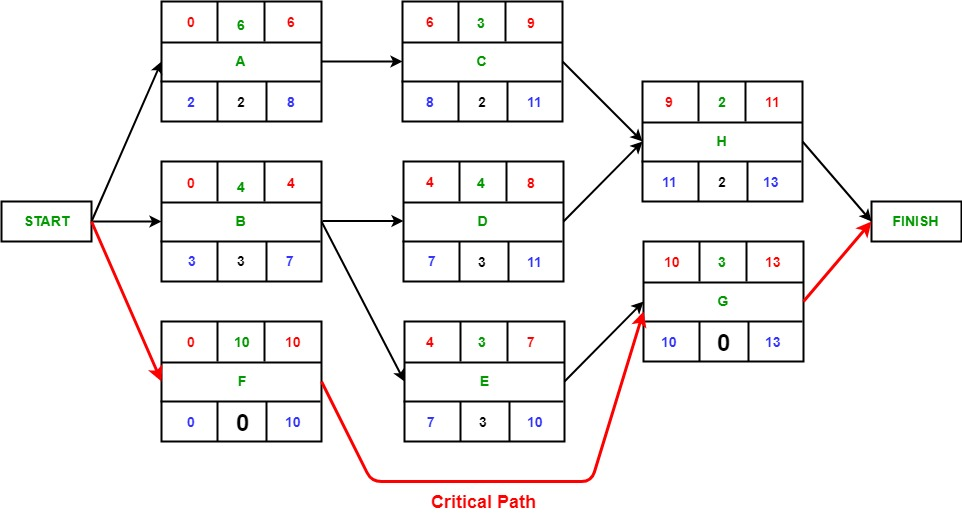My Personal Notes arrow_drop_upCheck out this Author's contributed articles.

If you like GeeksforGeeks and would like to contribute, you can also write an article using contribute.geeksforgeeks.org or mail your article to contribute@geeksforgeeks.org. See your article appearing on the GeeksforGeeks main page and help other Geeks.

Please Improve this article if you find anything incorrect by clicking on the "Improve Article" button below.

Improved By : Akanksha_Rai

Article Tags :

1

Please write to us at contribute@geeksforgeeks.org to report any issue with the above content.